开源免费多功能计算器 Qalculate ! 3.21.0 x64 中文版

2021年10月18日16:23:03评论22

软件介绍

Qalculate! 是一款多功能的跨平台桌面计算器应用。普通计算（如：货币转换和百分比计算）时简单易用，对于数理化问题而言功能丰富。其功能包扩海量自定义函数库、单位换算及计算、物理常数库、符号计算（包含积分和方程）、高精度计算、误差传递、区间算法、绘图以及用户友好界面（GTK+ and CLI）。

用户界面

• 使用 GTK+-3.0 实现用户图形界面;
• 灵活的表达式输入方式和单独显示结果;
• 显示计算结果精确与否;
• 可折叠下半按钮界面;
• 通过适用的菜单项快速进入高级设置;
• 显示计算历史记录;
• 可切换的传统计算按钮;
• 快捷对话框更容易使用和管理函数、变量和单位;
• 为函数描述和各参数项采用界面友好的对话框;
• 可创建/编辑函数、变量和单位;
• 可轻易编辑矩阵和向量;
• 简易的 gnuplot 界面;
• 进制转换采用不同界面;
• 元素周期表;
• 完备的基于界面的附加说明功能;
• 更多...

截图预览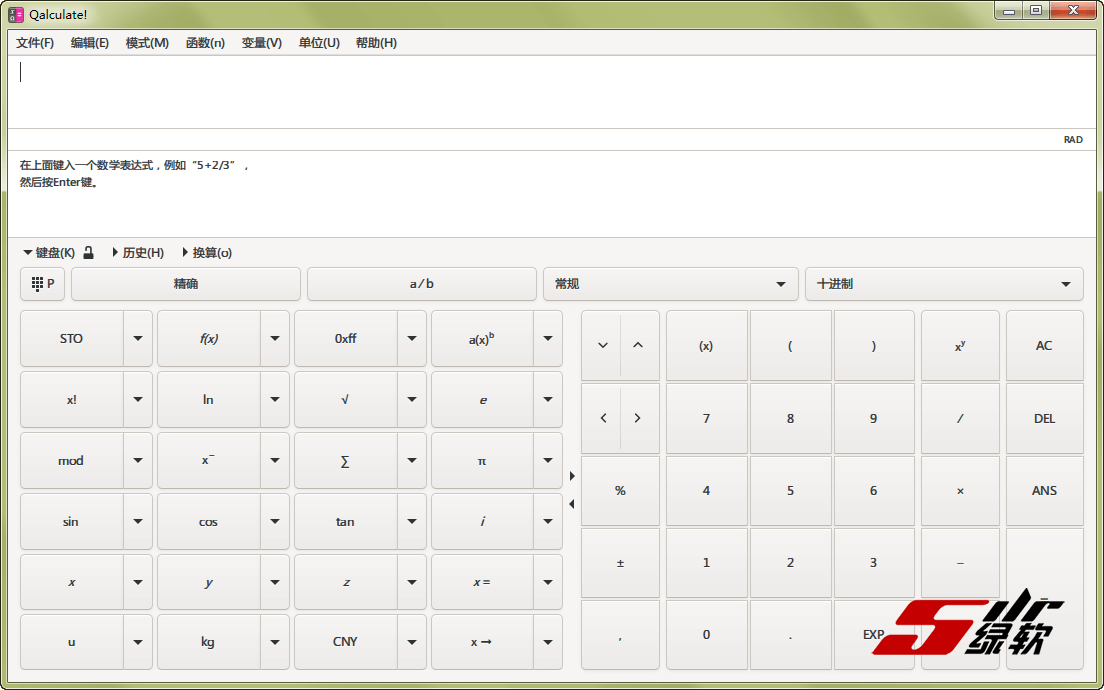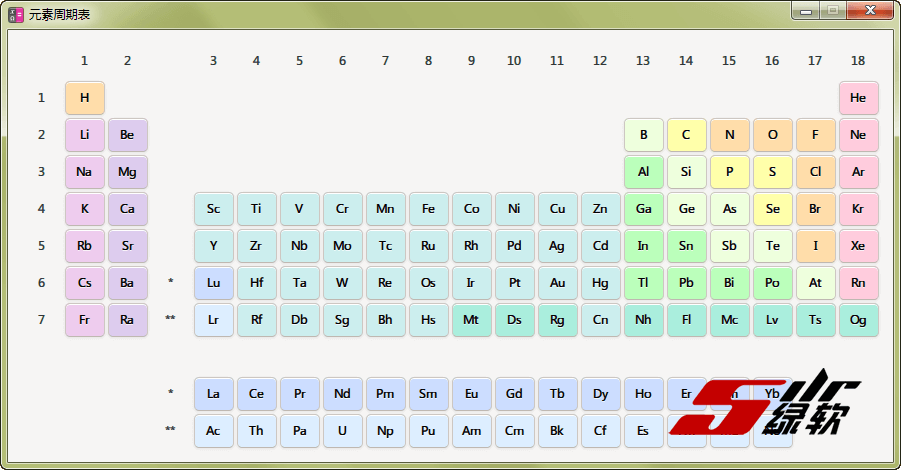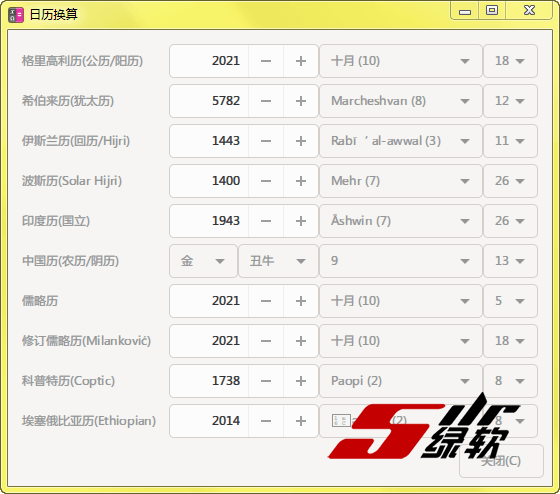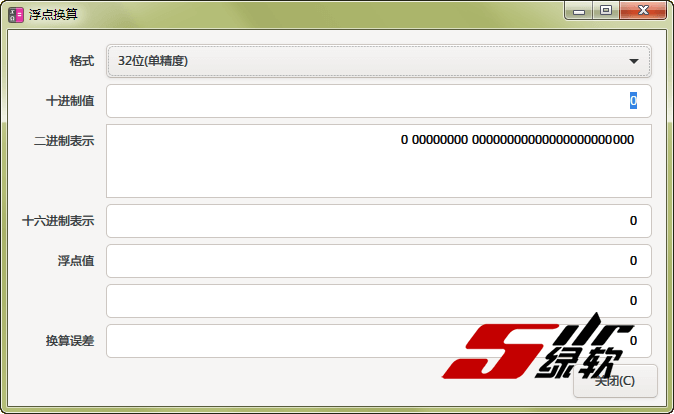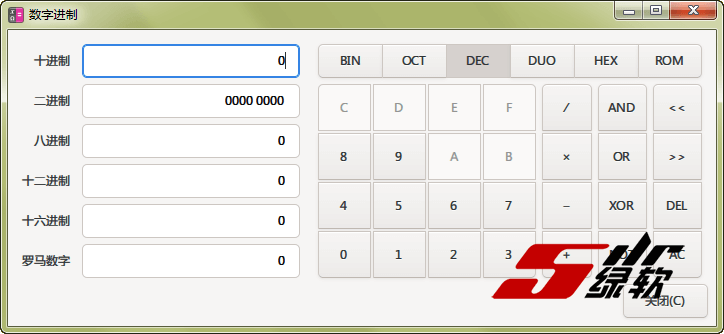功能特色

• 基本运算和操作符：+ - * / ^ E () && || ! < > >= <= != ~ & | << >>；
• 字符串的容错解析：log5/2.5(3)+(2(3+5 = ln(5)/(2.53)+23+5)；
• 表达式可以包含数字、函数、单位、变量、向量、矩阵及日期的任意组合；
• 支持复数和无穷量（∞）；
• 误差传递；
• 区间算法（用于确定有效数字或直接计算间隔的数）；
• 支持所有常用进制，以及负数、非整数根，六十进制数，时间格式和罗马数字；
• 可以禁用函数、变量、单位或未知数以避免混淆，例如：(a+b)^2 不表示 (are+barn)^2；
• 而是 ("a"+"b")^2；
• 可控的隐式乘法
• 支持矩阵、向量以及相关运算（行列式等）；
• 详细错误消息；
• 高精度计算；
• RPN 模式（逆波兰式计算）；

• 支持所有常用进制，以及负数、非整数根，六十进制数，时间格式和罗马数字；
• 许多自定义选项：精度、小数位数和乘号等；
• 精确（=）或近似（≈）；
• 分式：4/6*2=1.333... = 4/3=1+1/3；

• 例如：(x + y)^2 = x^2 + 2xy + y^2; 4 "apples" + 3 "oranges"；
• 因子分解和简化；
• 微分与积分；
• 能解大多数方程和不等式；
• 自定义假设得到不同结果，例如：ln(2x) = ln(2) + ln(x) 如果 x 为正；
• 能解大多数方程和不等式；

• 所有常用函数：sine, log, 等...例如：ln5 = 1.609; sqrt(tan(20)-5) = sqrt(-2.76283905578)；
• 大量统计、金融、几何及更多领域的函数（大约 200个）；
• If..then..else 函数，可选参数及可灵活创建更多特性的函数；
• 能解大多数方程和不等式；

• 支持所有国际单位制单位和前缀（包括二进制），也包括英制及其他单位系统；
• 自动转换：ft + yd + m = 2.2192 m；
• 隐式转换：5 m/s to mi/h = 11.18 miles/hour；
• 智能转换：可将 5 kg*m/s^2 转换为 5 newton；
• 误差传递；
• 区间算法（用于确定有效数字或直接计算间隔的数）；
• 容易创建、编辑或保存为标准 XML 文件；

• 基本常量：pi, e；
• 海量物理常量和元素；
• 可导入/导出为 CSV；
• 容易创建、编辑或存储为标准 XML 文件；
• 灵活，可以包含简单的数字、单位或者整个表达式；
• 在相似数据库结构中包含对象和属性关联的数据集；

• 采用gnuplot；
• 可绘制函数和数据（矩阵、向量）；
• 支持保存绘制图像为 PNG、postscript等...
• 多个自定义选项；

更新内容

qalculate.github.io

历史版本

• 本文原创发表于5ilr绿软！

• 除非特殊声明，本站文章均为原创，转载请务必保留本文链接！

https://www.5ilr.com/qalculate.html